Home > INT3 > Chapter 7 > Lesson 7.2.2 > Problem7-73

7-73.
1. Given that logx(2) = a, logx(5) = b, and logx(7) = c, write expressions using a, b, and/or c for each log expression below. Homework Help ✎

1. logx(10)

2. logx(49)

3. logx(50)

4. logx(56)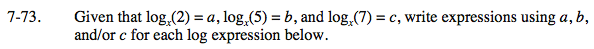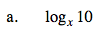$\log_{x}(2\cdot5)$

$\log_{x}(2)+\log_{x}(5)$

a + b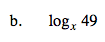2c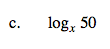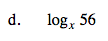3a + c